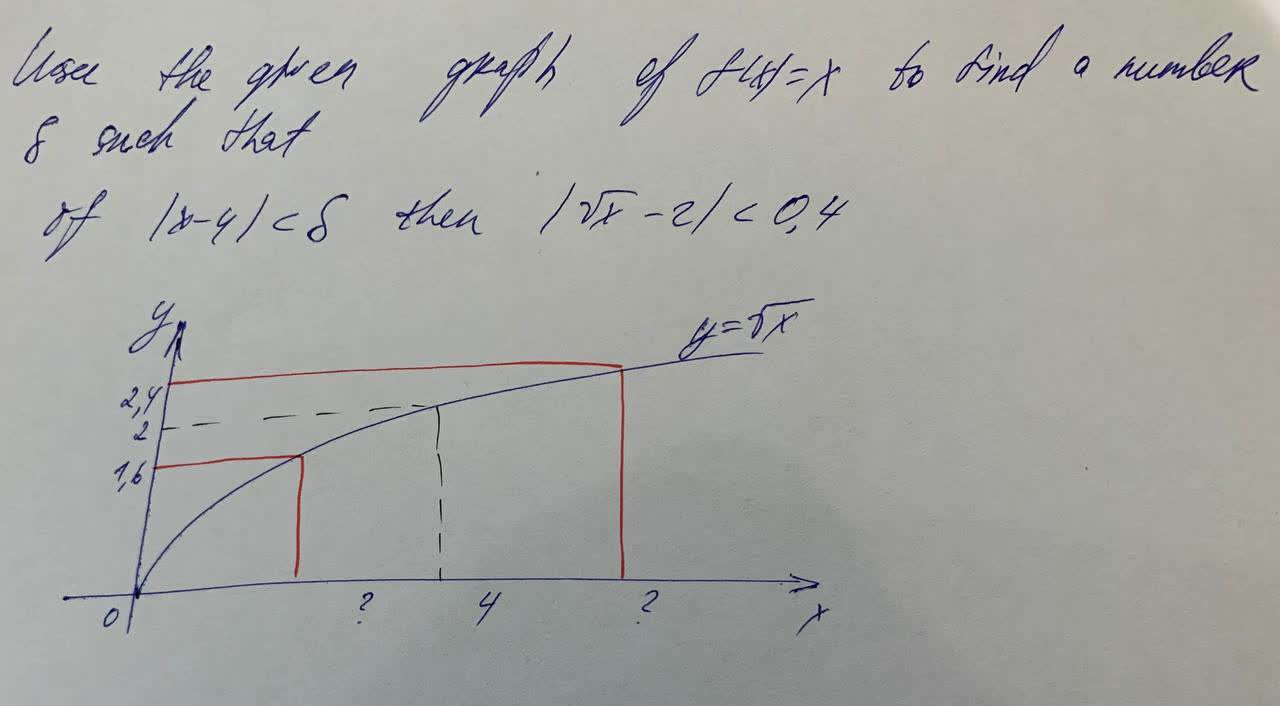Free answers to linear equations with graphs

Recent questions in Linear equations and graphspedzenekO 2021-07-30 Answered

Find equations of both lines through the point (2, −3) that are tangent to the parabola $$\displaystyle{y}={x}^{{2}}+{x}$$.nagasenaz 2021-07-05 Answered

Regarding linear equations with one independent variable, answer the following questions: a. What is the general form of such an equation? b. In your expression in part (a), which letters represent constants and which represent variables? c. In your expression in part (a), which letter represents the independent variable and which represents the dependent variable?Lewis Harvey 2021-07-05 Answered

Contain linear equations with constants in denominators. Solve each equation. $$\displaystyle\frac{{x}}{{3}}=\frac{{x}}{{2}}-{2}$$Ayaana Buck 2021-07-02 Answered

Contain linear equations with constants in denominators. Solve each equation. $$\displaystyle\frac{{x}}{{5}}-\frac{{1}}{{2}}=\frac{{x}}{{6}}$$postillan4 2021-06-30 Answered

You work at the local pool as a lifeguard and you also work in the snack bar. You earn $15 per hour lifeguarding and$8 per hour working in the snack bar. Last week you worked a total of 20 hours and earned \$202. a. Write an equation in standard form for the hours you worked. b. Write an equation in standard form for the money you earned. c. Graph both equations on the same coordinate plane. d. Determine how many hours you worked as a lifeguard and how many hours you worked in the snack bar. Explain your reasoning.ruigE 2021-06-29 Answered

Contain linear equations with constants in denominators. Solve each equation. $$\frac{3x}{5}-x=\frac{x}{10}-\frac{5}{2}$$Brittney Lord 2021-06-22 Answered

A student doing a Science Fair experiment put a hot bowl of soup in the refrigerator and checked the temperature of the soup every 2 minutes: a) Create a model describing how the soup cools. Be sure to look at the residuals to verify that your model is appropriate. b) Explain what the two values in the equation suggest about the soup. c) Estimate the temperature of the soup after 3 minutes. d) Estimate the temperature of the soup after 25 minutes. e) How much confidence do you place in those estimates? Why?Lipossig 2021-06-21 Answered

Contain linear equations with constants in denominators. Solve each equation. $$\displaystyle{20}-{\left(\frac{{x}}{{3}}\right)}=\frac{{x}}{{2}}$$mattgondek4 2021-06-20 Answered

$$\displaystyle{y}={3}+\sqrt{{{y}^{{2}}-{5}}}$$ankarskogC 2021-06-19 Answered

Contain linear equations with constants in denominators. Solve each equation. $$\displaystyle\frac{{x}}{{2}}={\left({3}\frac{{x}}{{4}}\right)}+{5}$$nitraiddQ 2021-06-13 Answered

$$\displaystyle{15}=-{6}-{\left({3}\frac{{p}}{{8}}\right)}$$sjeikdom0 2021-06-12 Answered

Find the point on the line $$y = 5x + 2$$ that is closest to the origin.Mylo O'Moore 2021-06-10 Answered

Use this graph to find a number of g such thatZoe Oneal 2021-06-10 Answered

Use the given graph of $$f(x) = x^2$$ to find a number $$(\delta)$$ such that if $$|x - 1| < (\delta)$$ then $$|x^2-1| < \frac{1}{2}$$ (Round your answer down to three decimal places.) $$(\delta) =$$Chardonnay Felix 2021-06-08 Answered

find the area of the largest rectangle that can be inscribed in the ellipse $$\frac{x^2}{a^2}+\frac{y^2}{b^2}=1$$Khadija Wells 2021-06-05 Answered

Contain linear equations with constants in denominators. Solve each equation. $$\displaystyle\frac{{x}}{{4}}={2}\frac{{{x}-{3}}}{{3}}$$midtlinjeg 2021-06-05 Answered

Find the area of the surface. $$z = \frac{2}{3}(x\frac{3}{2} + y\frac{3}{2})$$ , $$0 \leq x \leq 1, 0 \leq y \leq 1$$Emeli Hagan 2021-06-05 Answered

Where is the greatest integer function $$f(x) =[[x]]$$ not differentiable? Find a formula for f’ and sketch its graph.Isa Trevino 2021-06-02 Answered

Contain linear equations with constants in denominators. Solve each equation. $$\displaystyle\frac{{{x}+{1}}}{{3}}={5}-\frac{{{x}+{2}}}{{7}}$$Jaden Easton 2021-06-02 Answered

...